Home Page About Me Contact My Sound System Services Photo Album Work ExamplesOperational Amplifiers    The Op-amp is the fundamental circuit element for audio electronics. It makes all pre-amp circuitry possible in that it has extremely high input impedance, but can draw as much current as its power supply will allow at the same time. This IC (integrated circuit) uses the symbol of a triangle, combining two sources into one output, referenced to ground: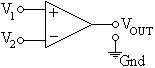The Op-amp I used in the graphic EQ I made from the ESP circuit board was a TL072. It packages two independent Op-amps in an 8-pin DIP (Dual In-line Package). It is smaller than a thumbnail and has a pin-out like so: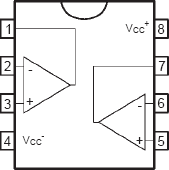Op-amps can be conceptualized by imagining that the input terminals are completely disconnected from anything. In reality, the input impedance is just so high that current is negligible (in the pico-amp range). The output voltage is derived from a series of transistors that take power from the supply rails (pins 4 and 8) and are controlled by the input voltage Vx. The system is basically a big dependent voltage source with value Vx and some multiplier A.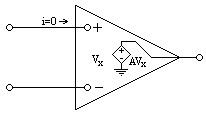The problem with Op-amps is that they have practically infinite gain. This sounds like a good thing, but "A" is completely unpredictable and can range from 1,000 to several millions, depending on the frequency of the input voltage. This is very bad for audio especially, so we need a solution. The trick is to fold the output back in on itself so the output is regulated.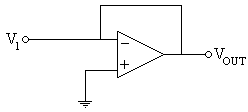Notice that as Vout rises, the inverting input is forced to rise with it. The gain is now 1. This is also not ideal in most cases. The next trick is to use some resistors to set the gain. The following configuration is an inverting amplifier, since the signal is present at the inverting input. Gain can be found after using Kirchoff's Current Law on the node between the resistors. When analyzing Op-amps, there are two conventions: Assume that the input current is zero. Inverting and non-inverting inputs have equal voltage.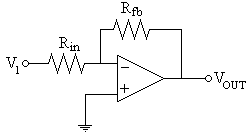(Vnode-V1)/Rin + (Vnode-Vout)/Rfb = 0 Vnode = 0 V1/Rin - Vout/Rfb = 0 Vout = -(Rfb/Rin)V1         The gain of the circuit is -(Rfb/Rin). The same process can be applied to any Op-amp configuration. Here are the most common configurations. InvertingVout = -(Rfb/Rin)V1 Non-inverting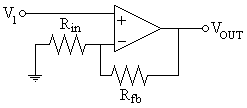Vout = (1+Rfb/Rin)V1           Unity-Gain (Buffer)Vout = V1 Summing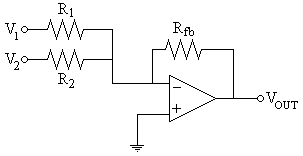Vout = -Rfb(V1/R1 + V2/R2) Differential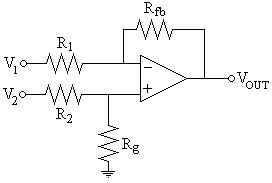Vout = Rfb(1 + R1/Rfb)V2/(R1(1 + R2/Rg)) - (Rfb/R1)V1

©2008